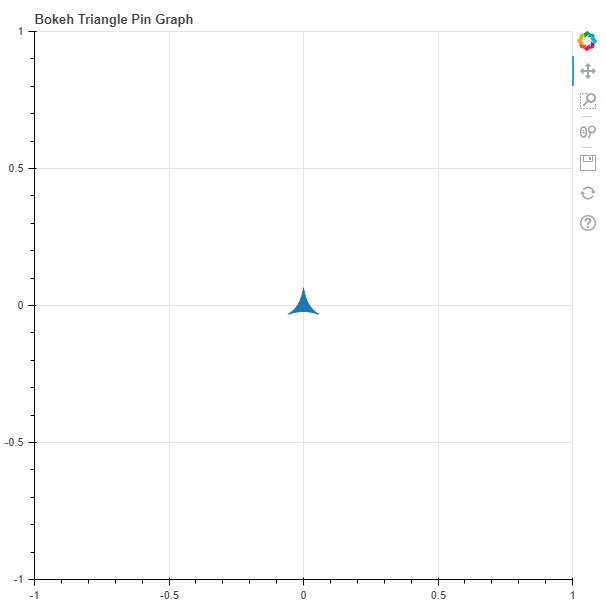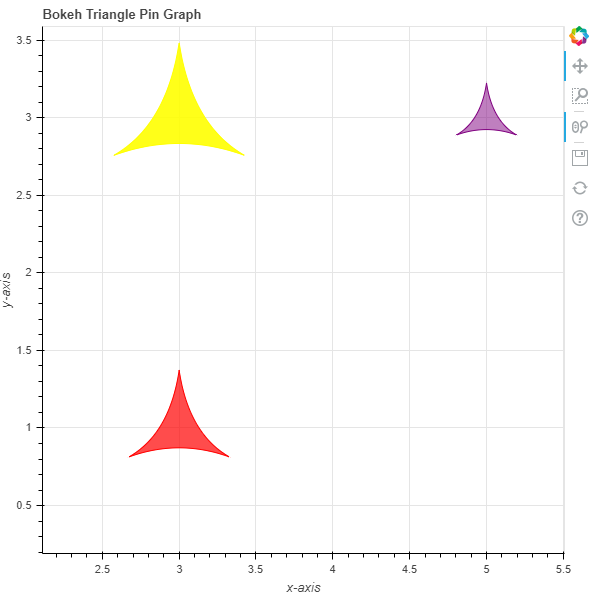# Python Bokeh – Plotting Triangle Pins on a Graph

• Last Updated : 10 Jul, 2020

Bokeh is a Python interactive data visualization. It renders its plots using HTML and JavaScript. It targets modern web browsers for presentation providing elegant, concise construction of novel graphics with high-performance interactivity.

Bokeh can be used to plot triangle pins on a graph. Plotting triangle pins on a graph can be done using the `triangle_pin()` method of the `plotting` module.

Attention geek! Strengthen your foundations with the Python Programming Foundation Course and learn the basics.

To begin with, your interview preparations Enhance your Data Structures concepts with the Python DS Course. And to begin with your Machine Learning Journey, join the Machine Learning - Basic Level Course

## plotting.figure.triangle_pin()

Syntax : triangle_pin(parameters)

Parameters :

• x : x-coordinates of the center of the triangle markers
• y : y-coordinates of the center of the triangle markers

Returns : an object of class `GlyphRenderer`

Example 1 : In this example we will be using the default values for plotting the graph.

 `# importing the modules``from` `bokeh.plotting ``import` `figure, output_file, show``        ` `# file to save the model``output_file(``"gfg.html"``)``        ` `# instantiating the figure object``graph ``=` `figure(title ``=` `"Bokeh Triangle Pin Graph"``)``      ` `# the points to be plotted``x ``=` `0``y ``=` `0``     ` `# plotting the graph``graph.triangle_pin(x, y, size ``=` `30``)``      ` `# displaying the model``show(graph)`

Output :Example 2 : In this example we will be plotting multiple triangle pins with various other parameters.

 `# importing the modules ``from` `bokeh.plotting ``import` `figure, output_file, show ``  ` `# file to save the model ``output_file(``"gfg.html"``) ``       ` `# instantiating the figure object ``graph ``=` `figure(title ``=` `"Bokeh Triangle Pin Graph"``) ``  ` `# name of the x-axis ``graph.xaxis.axis_label ``=` `"x-axis"``       ` `# name of the y-axis ``graph.yaxis.axis_label ``=` `"y-axis"``  ` `# points to be plotted``x ``=` `[``3``, ``3``, ``5``]``y ``=` `[``3``, ``1``, ``3``]``size ``=` `[``130``, ``100``, ``60``]``  ` `# color value of the triangles``color ``=` `[``"yellow"``, ``"red"``, ``"purple"``]``  ` `# fill alpha value of the triangles``fill_alpha ``=` `[``0.9``, ``0.7``, ``0.5``]``  ` `# plotting the graph ``graph.triangle_pin(x, y,``                   ``size ``=` `size,``                   ``color ``=` `color,``                   ``fill_alpha ``=` `fill_alpha) ``       ` `# displaying the model ``show(graph) `

Output :My Personal Notes arrow_drop_up